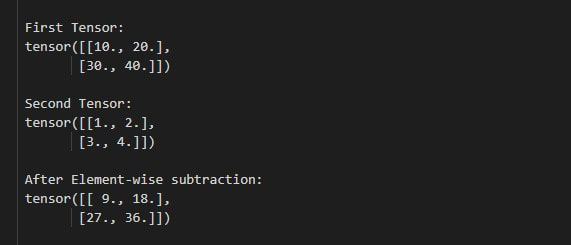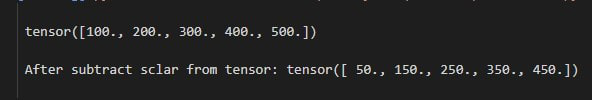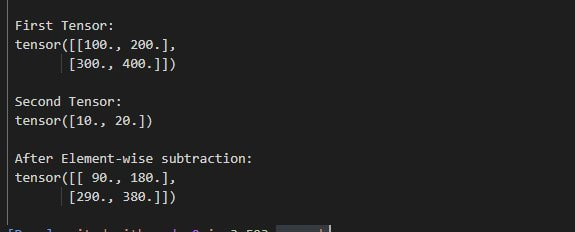Open in App
Not now

# How to perform element-wise subtraction on tensors in PyTorch?

• Last Updated : 24 Mar, 2022

In this article, we are going to understand how to perform element-wise subtraction on tensors in PyTorch in Python. We can perform element-wise subtraction using torch.sub() method.

torch.sub() method allows us to perform subtraction on the same or different dimensions of tensors. It takes two tensors as the inputs and returns a new tensor with the result (element-wise subtraction). If tensors are different in dimensions so it will return the higher dimension tensor. we can also subtract a scalar quantity with a tensor using torch.sub() function. We can use the below syntax to compute the element-wise subtraction.

Syntax: torch.sub(input, other, *, alpha=1, out=None)

Parameters:

• input: the input tensor.
• other: This is tensor or number to subtract from the input tensor.
• alpha (Number): the parameter is multiplier for other.
• out: it is the output tensor, This is optional parameter.

Return: it will returns a new modified tensor with element-wise subtraction of the tensor input by the tensor other.

Example 1:

The following program is to perform element-wise subtraction on two single dimension tensors.

## Python3

 `# import torch library``import` `torch` `# define two tensors``tens_1 ``=` `torch.Tensor([``10``, ``20``, ``30``, ``40``, ``50``])``tens_2 ``=` `torch.Tensor([``1``, ``2``, ``3``, ``4``, ``5``])` `# display tensors``print``(``" First Tensor: "``, tens_1)``print``(``" Second Tensor: "``, tens_2)` `# multiply tensors``tens ``=` `torch.sub(tens_1, tens_2)` `# display result after perform element wise``# subtraction``print``(``" After Element-wise subtraction: "``, tens)`

Output:Example 2:

The following program is to perform element-wise subtraction on two 2D tensors.

## Python3

 `# import torch library``import` `torch` `# define two tensors``tens_1 ``=` `torch.Tensor([[``10``, ``20``], [``30``, ``40``]])` `tens_2 ``=` `torch.Tensor([[``1``, ``2``], [``3``, ``4``]])` `# display tensors``print``(``"First Tensor:"``, tens_1)``print``(``"Second Tensor:"``, tens_2)` `# Subtract tensors``tens ``=` `torch.sub(tens_1, tens_2)` `# display result after perform element wise``# subtraction``print``(``"After Element-wise subtraction:"``, tens)`

Output:Example 3:

The following program is to know how to subtract a scalar quantity to a tensor.

## Python3

 `# import torch library``import` `torch` `# define a tensor``tens ``=` `torch.Tensor([``100``, ``200``, ``300``, ``400``, ``500``])` `# display tensors``print``(tens)` `# Subtract 50 from tensor``tens_result ``=` `torch.sub(tens, ``50``)` `# display result after subtract scalar from tensor``print``(``"After subtract scalar from tensor:"``, tens_result)`

Output:Example 4:

The following program is to understand how to perform element-wise subtraction on two different dimension tensors.

## Python3

 `# import torch library``import` `torch` `# define 2D tensor``tens_1 ``=` `torch.Tensor([[``100``, ``200``], [``300``, ``400``]])` `# define 1D tensor``tens_2 ``=` `torch.Tensor([``10``, ``20``])` `# display tensors``print``(``"First Tensor:"``, tens_1)``print``(``"Second Tensor:"``, tens_2)` `# Subtract tensors``tens ``=` `torch.sub(tens_1, tens_2)` `# display result after perform element wise subtraction``print``(``"After Element-wise subtraction:"``, tens)`

Output:My Personal Notes arrow_drop_up